Enthalpy of hydration of copper (II) sulfate You are provided with : - a sample of copper sulfate pentahydrate(CuSO 4.5H2O) - a sample of anhydrous copper sulfate (CuSO 4) - an electronic scales - 100 mL measuring cylinder - goggles - gloves - labcoat - a calorimeter - a thermometer The aim of this activity is to measure the enthalpy of hydration of copper sulfate using Hess's Law . The enthalpy of hydration is difficult to calculate as it is not possible to measure the chemical potential energy directly as the CuSO4.5H2O crystallizes.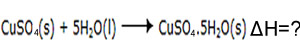We can calculate the ΔH of equation 3 if we know the ΔH of equation 1 and 2. You will perform this practical in two stages. First you will use the secondhand data below to determine the ΔH for reactions 1, below. Then you will use a calorimeter to measure the ΔH of equation 2. Click to go to this activity.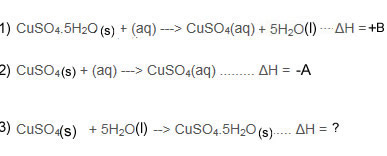Using Hess's Law you will then work out the ΔH of equation 3. This has two parts.   Part one - reverse equation 1 Part two - add it to equation 2.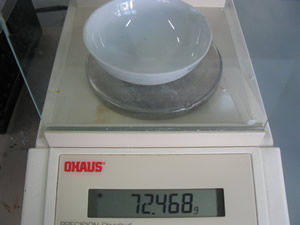Step 1 measure the mass of a crucible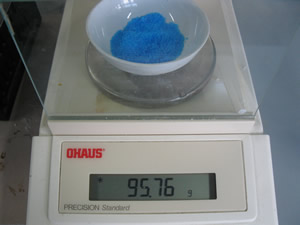Step 2 Measure accurately about 23.0 g of copper sulfate pentahydrate (CuSO4.5H2O )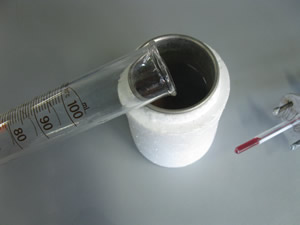Step 3 Add 100 mL of distilled water to the calorimeter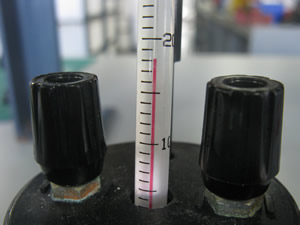Step 4 Measure the temperature of the water.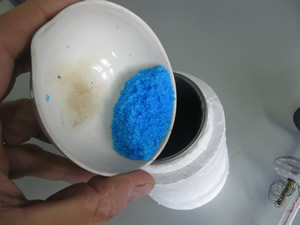Step 5 Add the CuSO4.5H2O to the water and seal the calorimeter. Stir the the water to allow for the CuSO4.5H2O to dissolve.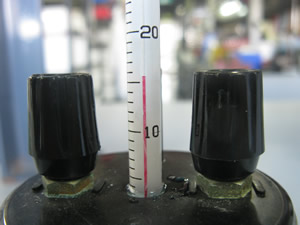Step 6 Record the final temperature of the water. Step 7 Calculate the ΔH. of the reaction below CuSO4 .5H2O (s) + (aq) => CuSO4(aq) + H2O(l)

Solution